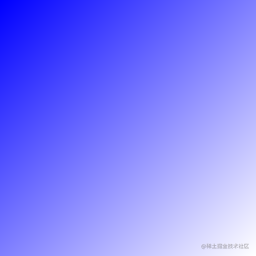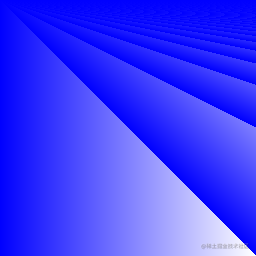# 基础模块

## 循环与函数

### 题目简述

$x$ 的平方根，从某个猜测的值 $z$ 开始，我们可以根据 $z^2$$x$ 的近似度来调整 $z$，产生一个更接近的猜测：

z -= (z*z - x) / (2*z)

### 解答

package main

import (
"fmt"
"math"
)

func Sqrt(x float64) float64 {
z := x / 2
d := 1.0
for math.Abs(d) > 1e-15 {
d = (z*z - x) / (2 * z)
z -= d
fmt.Println(z)
}
return z
}

func main() {
v:=2.0
fmt.Println("custom:",Sqrt(v))
fmt.Println("math:",math.Sqrt(v))
}

## 切片

### 解答

package main

import "golang.org/x/tour/pic"

func Pic(dx, dy int) [][]uint8 {
img := make([][]uint8, dy)
for y := range img {
img[y] = make([]uint8, dx)
for x := range img[y] {
img[y][x] = uint8((x + y) / 2)
}
}
return img
}

func main() {
pic.Show(Pic)
}(x + y) / 2 的图像x % (y + 1) 的图像

## 映射

### 解答

package main

import (
"golang.org/x/tour/wc"
"strings"
)

func WordCount(s string) map[string]int {
mp := make(map[string]int)
words := strings.Fields(s)
for _, v := range words {
mp[v]++
}
return mp
}

func main() {
wc.Test(WordCount)
}

strings.Fields() 会分割被空白字符围绕的字符串，然后返回一个字符串切片。遍历切片，统计字符串个数即可。这里可能会有人想用 ,ok 来检查字符串是否已经在映射中，然后分两种情况操作，这样也可以。但实际上， make 在创建映射时已经帮我们把空间初始化为零值，我们可以很方便地直接使用它。

## 斐波纳契闭包

### 解答

package main

import "fmt"

func fibonacci() func() int {
i := 0
a, b := 0, 1
return func() int {
if i == 0 {
i++
return 0
} else {
a, b = b, a+b
return a
}
}
}

func main() {
f := fibonacci()
for i := 0; i < 10; i++ {
fmt.Println(f())
}
}

func fibonacci() func() int {
a, b, c := 0, 0, 1
return func() int {
a, b, c = b, c, b+c
return a
}
}